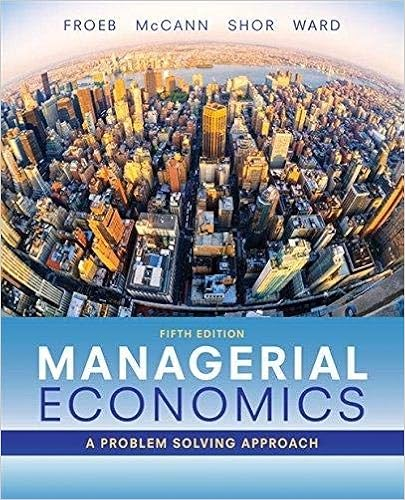# A 129 10 a business produces 5000 units per month it

• Test Prep
• 4
• 93% (184) 171 out of 184 people found this document helpful

This preview shows page 1 - 3 out of 4 pages.

##### We have textbook solutions for you!
The document you are viewing contains questions related to this textbook.The document you are viewing contains questions related to this textbook.
Chapter 6 / Exercise 6-1
Managerial Economics
Froeb/McCannExpert Verified
a. 1.29
10. A business produces 5,000 units per month. It spends \$12,000 on raw materials. It pays wages of \$20,000. Other costs include \$50,000 for rent, paid by the month. In order to break even, the selling price per unit will have to be:
##### We have textbook solutions for you!
The document you are viewing contains questions related to this textbook.The document you are viewing contains questions related to this textbook.
Chapter 6 / Exercise 6-1
Managerial Economics
Froeb/McCannExpert Verified
11. Use the table provided to answer the following question. If the firm hires five workers, the average cost equals: 12. An increase in the price of a complement shifts the demand curve to the: 13. An increase in the price of a substitute shifts the demand curve to the: 14. A buyer values a house at \$525,000 and a seller values the same house at \$485,000. If sales tax is 8% and is levied on the seller, then what would be the lowest price at which the seller would be willing to sell?
15. Peter's Pizzeria sells both pizzas and soda. It wants to increase the sales of its pizzas. Assuming that the pizza and the sodas are complements, which of these strategies can it employ?
16. The difference between the minimum price the producer is willing to accept and the price the producer actually receives for a product is referred to as:
17. Use the table provided to answer the following question.
•••